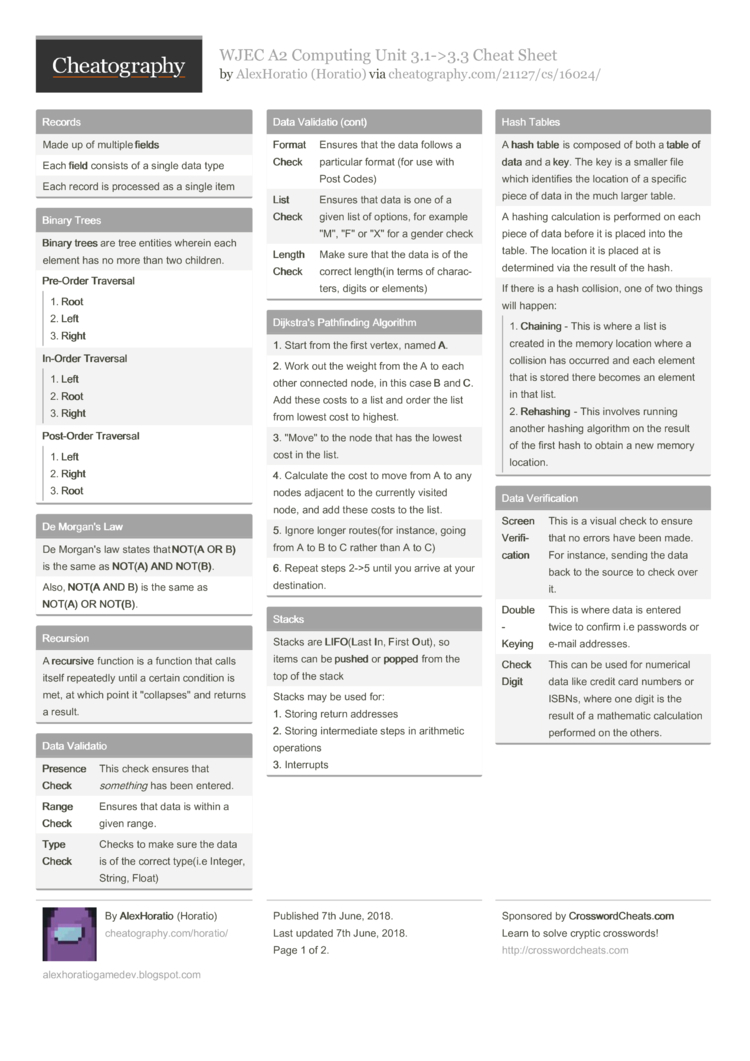# WJEC A2 Computing Unit 3.1->3.3 Cheat Sheet by Horatio

### Records

 Made up of multiple fields Each field consists of a single data type Each record is processed as a single item

### Binary Trees

 Binary trees are tree entities wherein each element has no more than two children. Pre-­Order Traver­sal1. Root 2. Left 3. Right In-Order Traver­sal1. Left 2. Root 3. Right Post­-Order Traver­sal1. Left 2. Right 3. Root

### De Morgan's Law

 De Morgan's law states that NOT(A OR B) is the same as NOT(A) AND NOT(B). Also, NOT(A AND B) is the same as NOT(A) OR NOT(B).

### Recursion

 A recu­rsive function is a function that calls itself repeatedly until a certain condition is met, at which point it "­col­lap­ses­" and returns a result.

### Data Validatio

 Presence Check This check ensures that somet­hing has been entered. Range Check Ensures that data is within a given range. Type Check Checks to make sure the data is of the correct type(i.e Integer, String, Float) Format Check Ensures that the data follows a particular format (for use with Post Codes) List Check Ensures that data is one of a given list of options, for example "­M", "­F" or "­X" for a gender check Length Check Make sure that the data is of the correct length(in terms of charac­ters, digits or elements)

### Dijkstra's Pathfi­nding Algorithm

 1. Start from the first vertex, named A. 2. Work out the weight from the A to each other connected node, in this case B and C. Add these costs to a list and order the list from lowest cost to highest. 3. "­Mov­e" to the node that has the lowest cost in the list. 4. Calculate the cost to move from A to any nodes adjacent to the currently visited node, and add these costs to the list. 5. Ignore longer routes(for instance, going from A to B to C rather than A to C) 6. Repeat steps 2->5 until you arrive at your destin­ation.

### Stacks

 Stacks are LIFO­(­L­ast In, First Out), so items can be pushed or popped from the top of the stack Stacks may be used for: 1. Storing return addresses 2. Storing interm­ediate steps in arithmetic operations 3. Interrupts

### Hash Tables

 A hash table is composed of both a table of data and a key. The key is a smaller file which identifies the location of a specific piece of data in the much larger table. A hashing calcul­ation is performed on each piece of data before it is placed into the table. The location it is placed at is determined via the result of the hash. If there is a hash collision, one of two things will happen:1. Chai­ning - This is where a list is created in the memory location where a collision has occurred and each element that is stored there becomes an element in that list. 2. Reha­shing - This involves running another hashing algorithm on the result of the first hash to obtain a new memory location.

### Data Verifi­cation

 Screen Verifi­cat­ion This is a visual check to ensure that no errors have been made. For instance, sending the data back to the source to check over it. Doub­le-­Key­ing This is where data is entered twice to confirm i.e passwords or e-mail addresses. Check Digit This can be used for numerical data like credit card numbers or ISBNs, where one digit is the result of a mathematic calcul­ation performed on the others.

### Lossy Compre­ssion

 Some of the data is "­l­ost­" during compre­ssion Lossy data types include JPEG, MPEG or MP3

### Queues

 Queues are FIFO­(­F­irst In, First Out). These would be used for 1. Holding tasks for the computer to run 2. A keypress buffer 3. Spooling output to a disk while waiting for a printer

### Graphs

 Graphs can relate any number of element to any other number of elements. A Weighted Graph is where there is a value on each edge which represents the "­cos­t" of traversing it. Graphs can either be dire­cted or undi­rec­ted.1. A dire­cted graph is a graph wherein there is a one-way relati­onship between each node. 2. An undi­rec­ted graph is a graph wherein the relati­onship is bidire­ctional between each node.

### Data Flow Diagrams

 What it looks like What it is Weird Double­-Square Lookin Thing External Entity Rounded Square Process Rectangle Missing the Right Edge Data Storage Arrow Represents the flow of data(s­hould be labelled)

### Big-O Notation

 Constant Time O(1) No matter how much data you give this algorithm, it will always execute in the same amount of time. Linear Time O(n) The time taken for the algorithm to run is directly propor­tional to the size of the input data. Poly­nomial Time O(n­2) The time taken increases propor­tional to the square(or cube, or any other power greater than one) of the input size. Expo­nential Time O(2­n) The time taken will double with every additional unit(or triple, etc- the 2 can be any number greater than one) of input data. Loga­rithmic Time O(Lo­g(n)) The time taken increases logari­thm­ically, so the rate of increasing time with respect to input size actually decreases.

### Lossless Compre­ssion

 No data is lost in the process, and the entire original file can be recons­tru­cted. Another type of lossless compre­ssion is via Dict­ionary Encodi­ng, where the most frequent elements of data are removed and instead replaced with a "­tok­en" that points to the piece of data somewhere else in the file to prevent needless data duplic­ation. Also in Huffman Coding, the most frequent elements of data are given the shortest token to maximize compre­ssion. One type of lossless compre­ssion is known as Run Length Encodi­ng, where strings of the same data(e.g, "­BBB­BBB­BB") are replaced with the number of times to place the data in a row when decomp­ressing it.(8B)2 Pages
//media.cheatography.com/storage/thumb/horatio_wjec-a2-computing-unit-3-1-3-3.750.jpg

PDF (recommended)